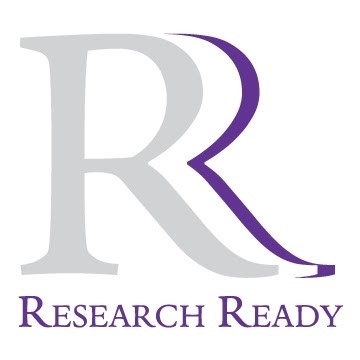# Data Analysis

The following module reviews qualitative and quantitative research approaches and commonly used research designs for each. The appropriate types of data analysis for each design are then outlined and the corresponding statistical tests are discussed.

#### Learning Objectives

• Identify appropriate data analysis for methods for each type of research design
• Identify which statistical tests could be applied to a particular data analysis method

There are two main approaches to a research problem - quantitative and qualitative methods. Quantitative methods are used to examine the relationship between variables with the primary goal being to analyze and represent that relationship mathematically through statistical analysis. This is the type of research approach most used in scientific research problems. Qualitative methods are chosen when the goal of the research problem is to examine, understand and describe a phenomenon. These methods are a common choice in social science research problems and are often used to study ideas, beliefs, human behaviors and other research questions that do not involve studying the relationship between variables.

Once the main approach to the research problem has been determined, there are several research designs for each type of approach that may be considered. The chart below breaks down the types of research design for each methodology. For more information regarding overall research methodology and design, refer to the Research Ready module on "Research Design". The purpose of this unit is to go one step farther and consider the primary types of data analysis methods that may be appropriate as well as the corresponding potential statistical test that could be applied. Each of the primary data analysis is discussed in additional detail below the chart.

GCU core research designs for dissertations in the Ed.D. program

 Methodology Design Primary Data Analysis* Selected Statistical Tests Quantitative Experimental/Quasi-Experimental Group comparison Independent-samples t-testPaired-sample t-testOne-way ANOVA Non-Experimental Descriptive Correlation/ RegressionGroup comparison Pearson correlationLinear regressionIndependent-samples t-testPaired-sample t-testSingle-sample t-testOne-way ANOVA Predictive Explanatory All Designs Sample or data characteristics Descriptive statistics Qualitative Ethnography Transcribing dataCoding dataCategorizing data N/A Phenomenology Case study All Designs Sample or data characteristics Descriptive statistics

*Primary data analysis does not preclude the data from being analyzed with other statistical approaches as needed within each of the research designs.

### Selected Statistical Tests for Primary Data Analysis Methods

Group Comparison Analyses:  There are several types of statistical tests that can be used to compare groups of participants or data sets. Following are some of the most common:

• T-Test: This is most basic form of group comparison and is used to compare to independent groups of participants and the data collected from those groups. The data sets are independent of one another and not related, therefore, this is sometimes referred to as the independent-sample t-test. An example would be to compare the test scores of students who took advantage of tutoring services with the test scores of students that do not use tutoring services.
• Paired-Sample T-Test: This test is used when the data sets are related in some way. This type of statistical test may be applied to look at the pre-test and post-test scores for a group of students taking a physics course. The pre-test and post-test scores are related in that they belong to the same person.
• Single-Sample T-Test: If the comparison needed is between a data set and fixed value, this test may be used. For example, final test scores in a chemistry course may be compared to a national average.
• ANOVA (Analysis of Variance): This is basic test that is used when comparing three or more sets of data in a way that requires several pair comparisons to be made. A researcher may need to compare the students test scores from 4 different elementary schools. Scores from each school will need to be compared with the scores from every other school.

Pearson Correlation Coefficient: This test is used to examine the linear correlation (dependence) between two variables, X and Y. The correlation draws a best fit through the data points created by X and Y and the coefficient is used to describe how far the points are from the best fit line, thereby, describes the level of correlation among data points. This type of statistical test may be used to examine the relationship between the amount of time spent studying and test scores.

Linear Regression: A linear regression test attempts to model the relationship between two variables by fitting a linear equation to the data. In this statistical test, one variable is an explanatory variable, and the other variable is a dependent variable. If the researcher were to examine the relationship between high school GPAs and University GPAs, the high school GPA would be the explanatory variable and the University GPA would be the dependent variable.

Descriptive Statistics: These types of basic statistical analyses attempt to quantify the main features of a collection of data and can be used on data from both qualitative and quantitative studies. Finding the mean, median, mode, and/or standard deviation of the data are the most common examples.

Choosing a Test and Data Analysis

The following 45-minute YouTube video describes the analyses discussed here in greater detail and provides examples of when a particular data analysis method may be helpful.

Devlin A. (2006) Research Methods. Thompson Wadsworth.
Dey, I. (2003). Qualitative data analysis: A user friendly guide for social scientists. Routledge.
Miles, M. B., & Huberman, A. M. (1994). Qualitative data analysis: An expanded sourcebook. Sage.
Judd, C. M., McClelland, G. H., & Ryan, C. S. (2009). Data analysis: A model comparison approach . Routledge/Taylor & Francis Group.
Quinn, G. G. P., & Keough, M. J. (2002). Experimental design and data analysis for biologists. Cambridge University Press.
Robson, C. (2002). Real world research: A resource for social scientists and practitioner-researchers (Vol. 2). Oxford: Blackwell.Viewed 11,864 times

Page Options